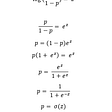# Introduction

In this blog post, I would like to continue my series on “building from scratch.” I will discuss a linear classifier called Logistic Regression. This blog post covers the following topics,

1. Basics of a classifier
2. Decision Boundaries
3. Maximum Likelihood Principle
4. Logistic Regression Equation
5. Logistic Regression Cost Function

After the discussion of the theoretical concepts we will dive into the code. So, without a further adieu let’s start the discussion with the basics of a classifier.

# Basics of a Classifier

A classifier is an estimator that assigns a class label to the input data point. Let’s take an example to understand it better. Let’s say we have images of animals and we want to associate them with their correct labels. For example, A dog’s image will be associated a label “Dog” or a cat’s image will be associated with a label “Cat” and the estimator which does this job can be called as a classifier. Classifiers are majorly of two types- A Binary Classifier & A Multi-Class Classifier. A binary classifier helps us associate data points with 2 labels while a multi-class classifier associates data points with multiple classes. Some examples of binary classifiers and multi-class classifiers are listed below:

Binary Classification Examples:

1. Spam/Ham mail classification
2. Fraudulent Transactions classification
3. Heart Disease classification

Multi-Class Classification Examples:

1. Animal Images Classification (Dog/Cat/Horse/Human)
2. Iris Flower Species Classification
3. MNIST Digit Classifier

Okay, so now we understand “a classifier” but what is a linear classifier? To understand a linear classifier, let’s look into the concept of the decision boundary.

# Decision Boundaries

Technically, a decision boundary is a region in space in which the output label of a classifier is ambiguous. Simply speaking if a data point lies on the decision boundary in space then it can belong to any of the classes. Intuitively, a decision boundary is a boundary that separates the classes in space. For example, if we have built a binary classifier which classifier data points into one of the two classes, then a decision boundary would be a boundary that separates the two classes. Data points lying on one side of the boundary would belong to one class and the points lying on the other side will belong to the other class. If this boundary is linear or a straight-line then it is known as a Linear Decision Boundary and the classifier that creates such a boundary is called a Linear Classifier. Logistic Regression is one such classifier. The below-attached image shows different types of decision boundaries generated using different models.

In the next, section we will talk about one of the most important topics in statistics and probability which is the basis of a lot of machine learning algorithms including the Logistic Regression, it’s called Maximum Likelihood Estimation.

# Maximum Likelihood Estimation

We are aware of various estimators or classifiers or models that give the best result on certain data but how are we able to know that a particular estimator is able to model the given data well. Every estimator has a certain set of parameters. For example, a Gaussian distribution has the mean & variance as the parameters. Now we have to determine the values of these parameters such that it helps us model the given data distribution. Using Maximum Likelihood Estimation we can calculate the ideal values of these parameters that can lead us to model the given data distribution.

Let us understand this with the help of an example, We have a data distribution that follows a Gaussian Distribution with parameters μ & σ which are unknown to us. We know that a Gaussian Distribution can be modeled using the following function,

Now, we can use the maximum likelihood estimation to estimate these parameters which would model the data. In other words, we need to find the parameters such that it would maximize the likelihood of this function modeling the given data. If you have studied calculus in mathematics then you must be aware of the fact that we can differentiate the function and equate it to zero to identify the points where it is maximized/minimized and use the double differential of the function to confirm whether the points obtained actually maximize or minimize the given function.

The above example, in fact, forms the basis of Mean Squared Error being selected as the loss function for Linear Regression.

Next, we look into the Logistic Regression algorithm while coding it side by side.

# Logistic Regression Equation

Before, going into the code let us understand the very basics of the Logistic Regression algorithm. Logistic Regression is a linear classifier that gives out the probabilities of a data point belonging to a particular class. The equation for the Logistic Regression is the same as the Linear Regression equation. Now the question arises how can a linear equation output probabilities. To convert the outputs of a linear equation into probabilities we use a mathematical function called sigmoid function. It is an “S” shaped function that limits the outputs of the linear equation between 0 & 1. Mathematically, it is given by,

Where e is the Euler’s constant. Next, we will look into the code of the sigmoid & logistic regression functions.

`# 0. Helper function: Sigmoiddef sigmoid(x):        '''    sigmoid(x) = 1 / (1 + e^(-x))    '''    return 1 / (1 + np.exp(-x))# 1. Hypothesis (Logistic Function)def hypothesis(x, theta):        # h(x) = sigmoid(X.theta)    z = np.dot(X, theta)        return sigmoid(z)`

Next, we will discuss the cost function used to measure the error while training the Logistic Regression algorithm.

# Logistic Regression Cost Function

While selecting the cost function we consider properties easy to optimize & should have no local minima because that can lead us to be stuck in a solution that is not optimal. In Linear Regression, we used Mean Squared Error as the Loss Function. However, in the case of a Logistic Regression doesn’t turn out to be a convex function i.e it has global as well as local minima. The below-attached image shows the difference between a convex & a non-convex function.

So, now we know that we cannot use Mean Squared Error as the cost function for Logistic Regression. So, what should be the cost function for training Logistic Regression? We use something known as Binary Cross-Entropy. It is given as,

Where yi is the actual class label and ŷi is the probability of the data point belonging to the first class. To learn more about the Binary Cross-Entropy refer to this blog post. Now we know the loss function for training the logistic regression model. Let’s try to code the same. The below-attached code snippet demonstrates this.

`# 2. Loss Function: Binary Cross Entropydef binary_cross_entropy(x, y, theta):        m, n = x.shape        # a. Compute the hypothesis    y_hat = hypothesis(x, theta)        # b. Compute the Binary Cross Entropy    loss = y * np.log(y_hat) + (1 - y) * np.log(1 - y_hat)        return - np.mean(loss)`

Now we have defined the Logistic Function, Binary Cross-Entropy Loss function, next we will see the algorithm to train the Logistic Regression model.

Just like Linear Regression, the Gradient Descent Algorithm can be used to train the Logistic Regression model & obtain the ideal parameters. I have explained the theory & the intuition of the Gradient Descent Algorithm in one of my blog posts. If you think that you need a refresher on it please refer to this blog post. The code for the Gradient Descent Algorithm can be found attached below.

`# 3. Compute the gradientdef gradient(x, y, theta):        # Compute hypothesis    y_hat = hypothesis(x, theta)        # Compute gradient    grad = np.dot( x.T, (y - y_hat))        return - grad / x.shape# 4. Gradient Descentdef gradient_descent(x, y, n_iter = 100, alpha = 0.1):        # a. Randomly initialise theta    m,n = x.shape    theta = np.zeros(shape = (n, ))        # List to store the error    error = []        # b. Perform the gradient descent    for i in range(n_iter):        '''        y_hat = hypothesis(x, theta)        print(y_hat, y_hat.shape)        '''                # b.1. Compute the loss        loss = binary_cross_entropy(x, y, theta)        error.append(loss)                # b.2. Copmute Gradient        grad = gradient(x, y, theta)                # b.3. Perform the update rule        theta = theta - alpha * grad            return theta, error`

Now, we have coded the entire algorithm from scratch let’s test its performance on a custom dataset and btw you can refer to the entire notebook on my Kaggle profile using this link.

# Testing the Algorithm

In this section, we test our Logistic Regression on a custom dataset & compare its performance with Scikit Learn’s version of Logistic Regression.

## 1. Make a custom dataset

We make a custom dataset using sklearn’s make_blobs function & visualize it using the seaborn library. The below code cell demonstrates the same.

`# 1. Create DatasetX, y = make_blobs(n_samples = 1000, n_features = 2, centers=2, random_state=0)dataset_array = np.concatenate((X, y.reshape(-1,1)), axis=1)# 2. Create a Dataframe of the arraydataset_df = pd.DataFrame(dataset_array, columns = ['Col 1', 'Col 2', 'Target'])# 3. plot the datasetsns.scatterplot(data=dataset_df, x='Col 1', y='Col 2', hue='Target')plt.xlabel("Column 1")plt.ylabel("Column 2")plt.show()`

## 2. Testing the Logistic Regression & Visualising the loss

Next, we test the algorithm & visualize the loss. The below-attached code cell demonstrates the following.

`X = dataset_df_copy.drop('Target', axis=1)y = dataset_df_copy['Target']theta, error = gradient_descent(X, y, 10000)# plot the errorplt.plot(error)plt.xlabel("Number of iterations")plt.ylabel("Error")plt.show()`

From the above plot, it can be observed that after ~2000 iterations the error starts to saturate and doesn’t decrease further. We can say that the algorithm has reached the minima. Next, we make the predictions & plot the decision boundary of the logistic regression.

## 3. Predictions & Decision Boundary

Next, we generate the decision boundary & visualize the extent of separation of the two classes by the boundary. Since the Logistic Regression is a linear model, the decision boundary will be a straight line. The following code cell demonstrates the same.

`# plot the dataset along with the decision boundary# Create Decision Boundaryx2_max, x2_min = X['Col 2'].max(), X['Col 2'].min()x1_max, x1_min = X['Col 1'].max(), X['Col 1'].min()x_vals = np.array([-2, 5])slope = - theta / thetaintercept = - theta / thetadecision_boundary = slope * x_vals + intercept# Plot the dataset with decision boundaryplt.figure(figsize=(12,8))sns.scatterplot(data=dataset_df, x='Col 1', y='Col 2', hue='Target')plt.plot(x_vals, decision_boundary, linestyle='--', color='black', label='Decision Boundary')plt.fill_between(x_vals, decision_boundary, x2_min-10, color='tab:orange', alpha=0.2)plt.fill_between(x_vals, decision_boundary, x2_max+10, color='tab:blue', alpha=0.2)plt.xlabel("Column 1")plt.ylabel("Column 2")plt.ylim(x2_min-1, x2_max)plt.xlim(x1_min, 5)plt.legend(loc='best')plt.show()`

From the above plot, it can be clearly observed that the Logistic Regression model is able to separate the two classes almost perfectly. Next, we see the performance of Scikit Learn’s Logistic Regression & compare it with our own.

## 4. Comparison with Scikit-Learn Logistic Regression

Next, we generate results on our custom dataset using Scikit-Learn’s Logistic Regression model & compare its performance with our own. The below code cell implements the same.

`# Import logistic regressionfrom sklearn.linear_model import LogisticRegression# Build the modellr = LogisticRegression()lr.fit(X.drop('Constant', axis=1), y)# Compute coeffecientstheta_sklearn = lr.coef_intercept_sklearn = lr.intercept_# Plot the Decision Boundary# plot the dataset along with the deicision boundary# Create Decision Boundaryx2_max, x2_min = X['Col 2'].max(), X['Col 2'].min()x1_max, x1_min = X['Col 1'].max(), X['Col 1'].min()x_vals = np.array([-2, 5])slope = - theta_sklearn / theta_sklearnintercept = - intercept_sklearn / theta_sklearndecision_boundary = slope * x_vals + intercept# Plot the dataset with decision bounddartplt.figure(figsize=(12,8))sns.scatterplot(data=dataset_df, x='Col 1', y='Col 2', hue='Target')plt.plot(x_vals, decision_boundary, linestyle='--', color='black', label='Decision Boundary')plt.fill_between(x_vals, decision_boundary, x2_min-10, color='tab:orange', alpha=0.2)plt.fill_between(x_vals, decision_boundary, x2_max+10, color='tab:blue', alpha=0.2)plt.xlabel("Column 1")plt.ylabel("Column 2")plt.ylim(x2_min-1, x1_max+4)plt.xlim(x1_min, 5)plt.legend(loc='best')plt.show()`

Next, we look into both the model’s parameters & compare the same.

`# Print the Custom Logistic Regression's resultsprint("Weights of variable given out by custom Logistic Regression")print("Col 1: {}".format(theta))print("Col 2: {}".format(theta))print("Intercept : {}".format(theta))print()print("Weights of variable given out by Sklearn's Logistic Regression")print("Col 1: {}".format(theta_sklearn))print("Col 2: {}".format(theta_sklearn))print("Intercept : {}".format(intercept_sklearn))`

Next, we use accuracy as a performance metric to quantify the model’s performance. We compute the accuracy score of both the custom as well as the sklearn’s Logistic Regression model & compare their performance.

`# Compute accuracy for both the models# 1. Custom Logistic Regressionpredictions_1 = np.round(hypothesis(X.drop('Constant', axis=1), theta))acc1 = np.sum(predictions_1 == y) / len(y) * 100# 2. Sklearn's Logistic Regressionpredictions_2 = lr.predict(X.drop('Constant', axis=1))acc2 = np.sum(predictions_2 == y) / len(y) * 100print("Accuracy of custom Logistic Regression Classifier: {}%".format(acc1))print("Accuracy of sklearn's Logistic Regression Classifier: {}%".format(acc2))`

Clearly, both the models are performing equally well. So, this was the entire comparison of both the Logistic Regression models. You can access the entire notebook here.

# Conclusion

I will conclude this blog post with a quick recap of what all we discussed. First, we learned about the basics of a classifier, then we learned about the Decision Boundaries followed by Maximum Likelihood Estimation. We started coding the Logistic Regression model & also discussed the cost function & gradient descent algorithm. Lastly, we compared the performance of our Logistic Regression with that of Python’s Scikit-Learn library.

I hope you found this blog post insightful. Please do share it with your friends & family and subscribe to my blog Keeping Up With Data Science for more informative content on Data Science straight to your inbox. You can reach out to me on Twitter & LinkedIn. I am quite active there & I will be happy to have a conversation with you. Please feel free to drop your feedback in the comments that helps me to improve the quality of my work. I will keep on sharing more content as I grow & mature as a Data Scientist. Until next time, Keep Hustling & Keep Up with Data Science. Happy Learning 🙂

--

--

--

## More from AIGuys

This publication is purely dedicated towards Data Science, AI and ML. From the state-of-the-art research papers to the most fundamental AI concepts.

## Your Transformer needs a Stabilizer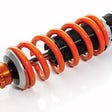## Trust Region Policy Optimisation(TRPO) — a policy-based Reinforcement Learning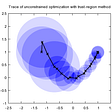## Managing Data Sources in Machine Learning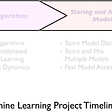## MICCAI 2021 HECKTOR challenge Study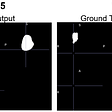## Exploring Deep Learning for Search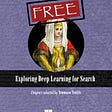## Learning Day 36: Implementing Autoencoder in Pytorch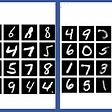## iOS 11: Are Apple’s new NLP capabilities game changers?## CoreML: The past, present, and future of Machine Learning in the Apple ecosystem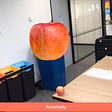## Chitwan Manchanda

Currently, working as a Data Science Intern at Editorialist YX. I like to write about Data Science and ML, checkout my work here bit.ly/KeepingUpWithDS

## Decision Tree From Sc## Machine Learning## Understanding the evaluation of Unsupervised Learning with K-Means## Classification algorithms 1: Logistic Regression Part-1